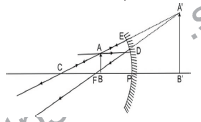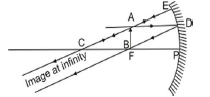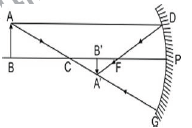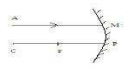# CBSE Class 10 Science Light Reflection and Refraction Assignment Set C

Read and download free pdf of CBSE Class 10 Physics Light Reflection and Refraction Assignment Set A. Get printable school Assignments for Class 10 Physics. Standard 10 students should practise questions and answers given here for Chapter 10 Light Reflection And Refraction Physics in Grade 10 which will help them to strengthen their understanding of all important topics. Students should also download free pdf of Printable Worksheets for Class 10 Physics prepared as per the latest books and syllabus issued by NCERT, CBSE, KVS and do problems daily to score better marks in tests and examinations

## Assignment for Class 10 Physics Chapter 10 Light Reflection And Refraction

Class 10 Physics students should refer to the following printable assignment in Pdf for Chapter 10 Light Reflection And Refraction in standard 10. This test paper with questions and answers for Grade 10 Physics will be very useful for exams and help you to score good marks

### Objective Questions

Question : Match the following with the correct response:

(1) Refraction                  (A) Bending of light
(2) Reflection                  (B) Velocity of light increases
(3) Rarer medium            (C) Bouncing back of light
(4) Denser medium         (D) Velocity of light decreases
a. 1-D, 2-A, 3-C, 4-B
b. 1-A, 2-C, 3-B, 4-D
c. 1-C, 2-B, 3-D, 4-A
d. 1-B, 2-D, 3-A, 4-C

Explanation: Refraction is the phenomenon of change in the direction of propagation of light when it passes from one transparent medium into another.
Reflection is the phenomenon of bouncing of light from the interface of an opaque medium. When a ray of light passes from a denser medium to a rarer medium, the velocity of light increases. When a ray of light passes from a rarer medium to a denser medium, the velocity of light decreases.

Question : Which of the following lens would you prefer to use while reading small letters found in a dictionary?
a. A convex lens of focal length 50 cm
b. A convex lens of focal length 5 cm
c. A concave lens of focal length 50 cm
d. A concave lens of focal length 5 cm

Explanation: A convex lens (converging lens) of focal length 5 cm should be used to read small letters in a dictionary. A convex lens of short focal length (5 cm) bends the light rays through large angles, by focussing them closer to the optical centre.

Question : An experiment to trace the path of a ray of light through a glass slab was performed by four students I, II, III and IV. They reported the following measurements of angle of incidence i, angle of refraction r and angle of emergence e. Which one of the students has performed the experiment correctly?a. III
b. I
c. II
d. IV

Explanation: It is most accurate since ∠e is (nearly) equal to ∠i. The correct value of ∠e = 60o.

### Light Reflection And Refraction Assertion Reason Class 10 Science

A. Both assertion and reason are true, and reason is the correct explanation of assertion.
B. Both assertion and reason are true, but reason is not the correct explanation of assertion.
C. Assertion is true but reason is false.
D. Assertion is false and reason is true.

Question. Assertion (A): Mirror Formula cannot be used for Plane Mirrors
Reason (R): Plane Mirror is a Spherical Mirror of Infinite Focal Length.

Question. Assertion(A): Virtual images are always erect.
Reason (R) : Virtual images are formed by converging lenses only.

Question. Assertion (A): Convex mirror is preferred for rear view mirrors in vehicles.
Reason (R) :The field view of a convex mirror is lesser than that of concave mirror.

Question. Assertion(A) : Concave mirrors are used as make-up mirrors.
Reason (R) : When the face is held within the focus of a concave mirror, then a diminished image of the face is seen in the concave mirror.

Question. Assertion (A): Light changes its speed when it passes from one medium to another.
Reason (R): When a ray travels from vacuum to a medium, then refractive index is known as absolute refractive index.

Question. Assertion (A): Large Concave mirrors are used to concentrate sunlight to produce heat in solar cookers.
Reason (R): Concave mirror converges the light rays falling on it to a point.

Question. Assertion (A): The angle of incidence for a ray of light having zero angle of reflection is two.
Reason (R): Refracting surfaces follow Snell’s Law.

Question. Assertion (A) : It is impossible to see virtual image with our naked Eye.
Reason (R) : The rays do not actually emanate from a virtual image.

Question. Assertion(A): Light travels faster in water than air
Reason (R): Water is denser than Air.

Question : Define one dioptre of power of a lens?
Answer : Power of a lens is one dioptre if focal length of a lens is 1m

Question : What is the name given to change of path of light with change of medium?
Answer : Refraction can change path of light with change of medium

Question : What is silvering of mirror?
Answer : Silvering of mirror means coating the surface of mirror with a thin layer of silver, aluminium or some other shiny, opaque material.

Question : Which lens bends a light ray more or less with a shorter or with longer focal length?
Answer : The lens with the shorter focal length bends the light more.

Question : Name some phenomenon associated with light during image formation by mirrors.

Question : Give the relation between focal length and radius of curvature.

Question : State lens formula and write it mathematically.
Answer : The relationship between object distance (u), image distance (v), and focal length of lens is known as lens formula. It is given by 1/v - 1/u = 1/f

Question : Define refraction of light.
Answer : The change in direction of light, when it travels from one medium to another medium is called refraction of light.

Question : Define lateral displacement.
Answer : Lateral displacement is the perpendicular distance between the incident ray and the emergent ray.

Question : Give uses of convex mirror.
Answer : (a) Used as rear view mirror in vehicles.
(b) Used to see full length image of a tall building.

Question : State laws of reflection.
Answer : Incident ray, reflected ray and normal at the point of incidence all lie in the same plane. The angle of incidence is equal to the angle of reflection.

Question : What are spherical mirrors?
Answer : Mirrors whose reflecting surface are part of a sphere are called spherical mirrors.

Question : What are the properties of image formed by a plane mirror?
Answer : Image is virtual and erect.
• Size of the image is equal to that of object
• Image is laterally inverted.
• The image formed by a plane mirror is always at the same distance as the object is in front of it.

Question : Define pole, centre of curvature, radius of curvature, principal axis, aperture, focus and focal length of a spherical mirror.
Answer : Pole: the centre of reflecting surface. It is represented by letter P. Centre of Curvature: The centre of the sphere of which the mirror forms the part.
Represented by “C”.
Radius of Curvature: The radius of the sphere of which the mirror forms the part.
Represented by “R”.
Principal axis: The straight line joining the pole (P) and the centre of curvature. It is normal to the mirror at its pole.
Aperture: The diameter of the spherical mirror is called its aperture. The reflecting surface of the mirror.
Focus: The point of the principal axis at which the rays parallel to principal axis meet (concave mirror) or appear to meet (convex mirror) after reflection. Represented by F.
Focal Length: The distance between the pole and the principal focus of a spherical mirror is called focal length. Represented by f.

Question : Define refractive index.
Answer : Refractive index is defined as the ratio of speed of light in medium 1 to the speed of light in medium 2 and is represented as n21 and is read as refractive index of medium 2 with respect to medium 1.
n21 = speed of light in medium 1/speed of light in medium 2.

Question : Define incident ray, reflected ray, normal ray, angle of incidence and reflection.
Answer : Incident ray – light which falls on the mirror/ polished surface is called incident ray.
Reflected ray – ray of light which goes back in the same medium after striking the surface is called reflected ray.
Normal – the perpendicular drawn to the reflecting surface is called normal at that point.
Angle of incidence – the angle between the incident ray and the normal is known angle of incidence.
Angle of reflection – the angle between reflected ray and the normal is known angle of reflection.

Question : State laws of refraction.
Answer : The ratio of sin of angle of incidence to the sin of angle of refraction for a light of given colour and for a given pair of media is constant. This is called Snell’s law.
i.e., sin i / sin r = Constant
The incident ray, refracted ray and the normal at the point of incidence lie on the same plane.

Question : State mirror formula and write it mathematically.
Answer : The relation between focal length of mirror, distance of the object and distance of the
image is known as mirror formula. It is given by
1/u + 1/v = 1/f
u = Image distance
ν = Object distance
f = Focal length

Question : Define magnification of mirror.
Answer : The ratio of height of the image to the height of the object is called magnification. It is represented by ‘m’.
m = Height of image (h′)/Height of object (h) = –v/u
Magnification of real image is negative and of virtual image is positive.

Question : What is the relation between optical density, refractive index and speed of light?
Answer : The medium with higher refractive index in which speed of light is less is known as optically denser medium and the medium with lower refractive index in which the speed of light is more is known as optically rarer medium.

Question : Define magnification of lens.
Answer : Magnification (m) = Height of image (h) / Height of object (h) = ν/u
For convex lens ‘m’ can be more than, less than or equal to one.
For concave lens ‘m’ is less than one.

Question : Define centre of curvature, principal axis, optical centre, aperture, focus and focal length for a lens.
Answer : (a) Centre of curvature: It is the centre of the spheres of which the each surface of the lens forms a part. Represented by C or 2f.
(b) Principal axis: An imaginary straight line passing through the two centres of curvatures.
(c) Optical centre: It is the central point of the lens. Represented by O.
(d) Aperture: It is the diameter of circular outline of a spherical lens.
(e) Focus: The point at which rays of light parallel to principal axis converges (convex lens) or appears to diverge (concave lens) after refraction. Represented by F.
(f) Focal length: The distance between focus and optical centre is called focal length. It is represented by f.

Question : With respect to air the refractive index of ice is 1.31 and that of rock salt is 1.54.
Calculate the refractive index of rock salt with respect to ice?Question : When a plane mirror is placed horizontally on the levelled ground at a distance of 40 m from the foot of a tower, the top of the tower and its image in the mirror subtend an angle of 90° at the eye. What is the height of the tower?From the picture, we see that the complementary angle of depression of the light ray is 45°.
So, if AB is the tower, and CB is the distance between the tower and the mirror, then, From the figure it is clear that tan 45o = h /40 (where h = AB is the height of tower)
1 = h/40
h = 40 cm

Question :  Form the image in case an object is moved from infinity to the concave mirror.
Answer :  Images formed by a concave mirror.
Object at Infinity. Two cases arise :
i. Object between F and P. A ray AD from A goes parallel to principal axis after reflection passes through F (rule 1). Another ray AE striking the mirror normally through C is reflected back (rule 3). They form virtual image of the object behind the mirror. The image is erect and enlarged.Object between F and P. An erect, enlarged, virtual image is formed behind the mirror.
Images Formed by a Concave mirror
ii. Object at F. A ray AD parallel to principal axis passes through F. Another ray AE strikes the mirror normally at E is reflected back as it passes through C (Rule 3).
They form image of object AB at infinity. The image is very much enlarged and is real and inverted.Object at E. Real, inverted, extremely enlarged image is formed at infinity.

iii. Object between F and C (f and 2f) A ray AD from object going parallel to principal axis is reflected towards F (Rule 1). Another ray AE through C is reflected back (Rule 3) forming image of A at A'. Similarly image of B is formed at B'. Image is real, inverted, enlarged and beyond C (2f) i.e. as shown in fig.iv. Object at C i.e. at 2f. A ray AD from A parallel to principal axis after reflection from mirror passes through F (Rule 1). Another ray AD' from A through F, goes parallel to principal axis i.e. towards D'A' (rule 2) forming real, inverted image of AB at C i.e. at 2f. The image is of the same size as the object.Object at C, Image is also at C. It is real.

v. Object beyond C. A ray AD from A parallel to principal axis after reflection passes through F (Rule 1), Another ray from A through C, ray AG is reflected back along the same path (Rule 3), forming real, diminished, inverted image of AB is formed at A'B', between F and C.Object beyond C, a real, inverted diminished image between F and C, inverted at C and is of same size as that of object.

vi. When mirror is inclined so that the rays strike the mirror obliquely. The ray AB passing through F after reflection goes parallel to principal axis towards BA' (Rule 2). Another ray DE through C striking the mirror at E is reflected back. The two form an image at A' Image is real, inverted, extremely diminished and at F.Object at infinity, image at F. It is real, inverted, very much diminished.

vii. When mirror is in parallel plane to the object. In such a case, rays from infinity come parallel to principal axis. After reflection they pass through principal focus F (Rule 1). Image is extremely small, it is real, inverted and at principal focus.Question : Name the type of mirror used in the following situations:
(i) Rear view mirror in vehicles
(ii) Solar furnace
(iii) Torch
(iv) Solar cooker
(v) To get the full length image of tall building.
Answer : (i) Rear view mirror in vehicles – convex mirror as it gives virtual image, diminished and cover the wider view.
(ii) Solar furnace – concave mirror to concentrate all parallel beam of light.
(iii) Torch – concave mirror is used.
(iv) Solar cooker – concave mirror is used to concentrate the heat rays at a point.
(v) Convex mirror is used to view a full length tall building.

### HOTS Questions

Question. Nidhi wanted the image of her pencil to be double the size of its original size. Name the mirror used for getting such image.

Question. An incident ray makes an angle of 60° with the mirror. What is the angle of reflection?

Question. What are the two types of reflection?
Answer : (i) Regular (ii) Irregular

Question. Give characteristics of image formed by plane mirror
Answer : • Image is virtual and erect.
• Size is same as of the object.
• It is formed at same distance.

Question. Name two types of spherical mirror.
• Convex mirror

Question. Give uses of convex mirror.
Answer : • It is used as the rear view mirror in cars.
• It is used in street lights as it diverge the light over larger area.

Question. Why do we prefer a convex mirror as a rear view mirror in vehicles?
Answer : Convex mirrors are used as rear view mirror in cars because it produces erect and diminished image of the traffic behind the vehicle. It also gives a wider view.

Question. The magnification produced by a plane mirror is +1. What does this mean?
Answer : This means that the size of the image is equal to the size of the object.

### VBQs

Question. In a small town fair Akshay took his friend and showed him a mirror in which his image showed upper half body very fat and lower body very thin. Akshay’s friend got upset but
Akshay explained him by showing his similar image in the mirror.

(a) Name two mirrors used in this fair shop.
(b) Name the mirror in which the size of image is small.
(c) What value of Akshay is reflected?

Answer : (a) Concave and convex mirror.
(b) Convex mirror gives small size image.
(c) Akshay showed compassion and empathy.

Q1 A ray of light AM is incident on a spherical mirror as shown in the diagram. Redraw the diagram and show the path of reflected ray.Q2 Explain why a ray of light passing through the centre of curvature of a concave mirror gets reflected along the same path?
Q3 What is the radius of plane mirror?
Q4 Why do we use convex surface for side view mirror?
Q5 Relate the focal length ‘f ‘ and the radius of curvature ‘R’.
Q6 Which kind of mirror is used in the headlights of a motor car and why?
Q7 What is focal length of a plane mirror?
Q8 A concave mirror is placed in water. Will there be any change in the focal length? Give reasons.
Q9 Name the type of mirror which always forms a virtual and diminished image.
Q10 A ray of light LM is incident on a mirror as shown in the figure. The angle of incidence for the ray is the angle between it and the line joining the other points in the figure. Name these two points.Q12 Can a virtual image be photographed?
Q13 Locate the position of image formed by a concave mirror if the object is at 2F or C.
Q14 Find the focal length of a convex mirror whose radius of curvature is 32cm.

Please click the below link to access CBSE Class 10 Physics Light Assignment Set A

 CBSE Class 10 Science Chemical Reactions and Equations Assignment
 CBSE Class 10 Science Acids Bases and Salts Assignment Set A CBSE Class 10 Science Acids Bases and Salts Assignment Set B CBSE Class 10 Science Acids Bases and Salts Assignment Set C
 CBSE Class 10 Science Metals and Non Metals Assignment Set A CBSE Class 10 Science Metals and Non Metals Assignment Set B
 CBSE Class 10 Science Carbon and its compounds Assignment Set A CBSE Class 10 Science Carbon and its compounds Assignment Set B CBSE Class 10 Science Carbon and its compounds Assignment Set C CBSE Class 10 Science Carbon and its compounds Assignment Set D CBSE Class 10 Science Carbon and its compounds Assignment Set E
 CBSE Class 10 Science Periodic Classification Of Elements Assignment Set A CBSE Class 10 Science Periodic Classification Of Elements Assignment Set B CBSE Class 10 Science Periodic Classification Of Elements Assignment Set C
 CBSE Class 10 Science Biology Life Process Respiration Assignment CBSE Class 10 Science Biology Nutrition Assignment CBSE Class 10 Science Biology Respiration Assignment CBSE Class 10 Science Biology Transport Enrichment Assignment CBSE Class 10 Science Biology Transportation Assignment CBSE Class 10 Science Life Processes Assignment Set A CBSE Class 10 Science Life Processes Assignment Set B CBSE Class 10 Science Life Processes Assignment Set C
 CBSE Class 10 Science Control and Coordination Assignment Set A CBSE Class 10 Science Control and Coordination Assignment Set B CBSE Class 10 Science Control and Coordination Assignment Set C CBSE Class 10 Science Control and Coordination Assignment Set D
 CBSE Class 10 Science How do Organisms Reproduce Assignment Set A CBSE Class 10 Science How do Organisms Reproduce Assignment Set B
 CBSE Class 10 Science Heredity and Evolution Assignment Set A CBSE Class 10 Science Heredity and Evolution Assignment Set B CBSE Class 10 Science Heredity and Evolution Assignment Set C
 CBSE Class 10 Science Light Reflection and Refraction Assignment Set A CBSE Class 10 Science Light Reflection and Refraction Assignment Set B CBSE Class 10 Science Light Reflection and Refraction Assignment Set C CBSE Class 10 Science Light Reflection and Refraction Assignment Set D CBSE Class 10 Science Light Reflection and Refraction Assignment Set E CBSE Class 10 Science Light Reflection and Refraction Assignment Set F
 CBSE Class 10 Science Human Eye and Colorful World Assignment Set A CBSE Class 10 Science Human Eye and Colorful World Assignment Set B CBSE Class 10 Science Human Eye and Colorful World Assignment Set C
 CBSE Class 10 Physics Electricity Assignment Set A CBSE Class 10 Physics Electricity Assignment Set B CBSE Class 10 Physics Electricity Assignment Set C CBSE Class 10 Science Electricity Assignment Set A CBSE Class 10 Science Electricity Assignment Set B CBSE Class 10 Science Electricity Assignment Set C
 CBSE Class 10 Science Magnetic Effects of Electric Current Assignment Set A CBSE Class 10 Science Magnetic Effects of Electric Current Assignment Set B CBSE Class 10 Science Magnetic Effects of Electric Current Assignment Set C CBSE Class 10 Science Magnetic Effects of Electric Current Assignment Set D
 CBSE Class 10 Science Sources of Energy Assignment Set A CBSE Class 10 Science Sources of Energy Assignment Set B CBSE Class 10 Science Sources of Energy Assignment Set C CBSE Class 10 Science Sources of Energy Assignment Set D
 CBSE Class 10 Science Our Environment Assignment Set A CBSE Class 10 Science Our Environment Assignment Set B CBSE Class 10 Science Our Environment Assignment Set C
 CBSE Class 10 Science Sustainable Management of Natural Resources Assignment Set A CBSE Class 10 Science Sustainable Management of Natural Resources Assignment Set B CBSE Class 10 Science Sustainable Management of Natural Resources Assignment Set C
 CBSE Class 10 Physics Revision Assignment Set A CBSE Class 10 Physics Revision Assignment Set B CBSE Class 10 Physics Revision Assignment Set C CBSE Class 10 Physics Revision Assignment Set D CBSE Class 10 Physics Revision Assignment Set E CBSE Class 10 Physics Revision Assignment Set F CBSE Class 10 Physics Revision Assignment Set G CBSE Class 10 Physics Revision Assignment Set H
 CBSE Class 10 Science Assignment CBSE Class 10 Science Assignments Collection CBSE Class 10 Science Energy Crossword Puzzle Assignment
 CBSE Class 10 Physics Question Bank CBSE Class 10 Physics Tungsten Assignment
 CBSE Class 10 Science Chemistry Cleaning Capacity of Soap Assignment CBSE Class 10 Science Chemistry Determination of pH Assignment CBSE Class 10 Science Chemistry Different Types of Chemical Reactions Assignment CBSE Class 10 Science Chemistry Displacement Reaction and Reactivity Series Assignment CBSE Class 10 Science Chemistry Properties of Acetic Acid Assignment CBSE Class 10 Science Chemistry Saponification Preparation of Soap Assignment CBSE Class 10 Science Chemistry Study of properties of HCI Acid and NaOH Assignment

Tags